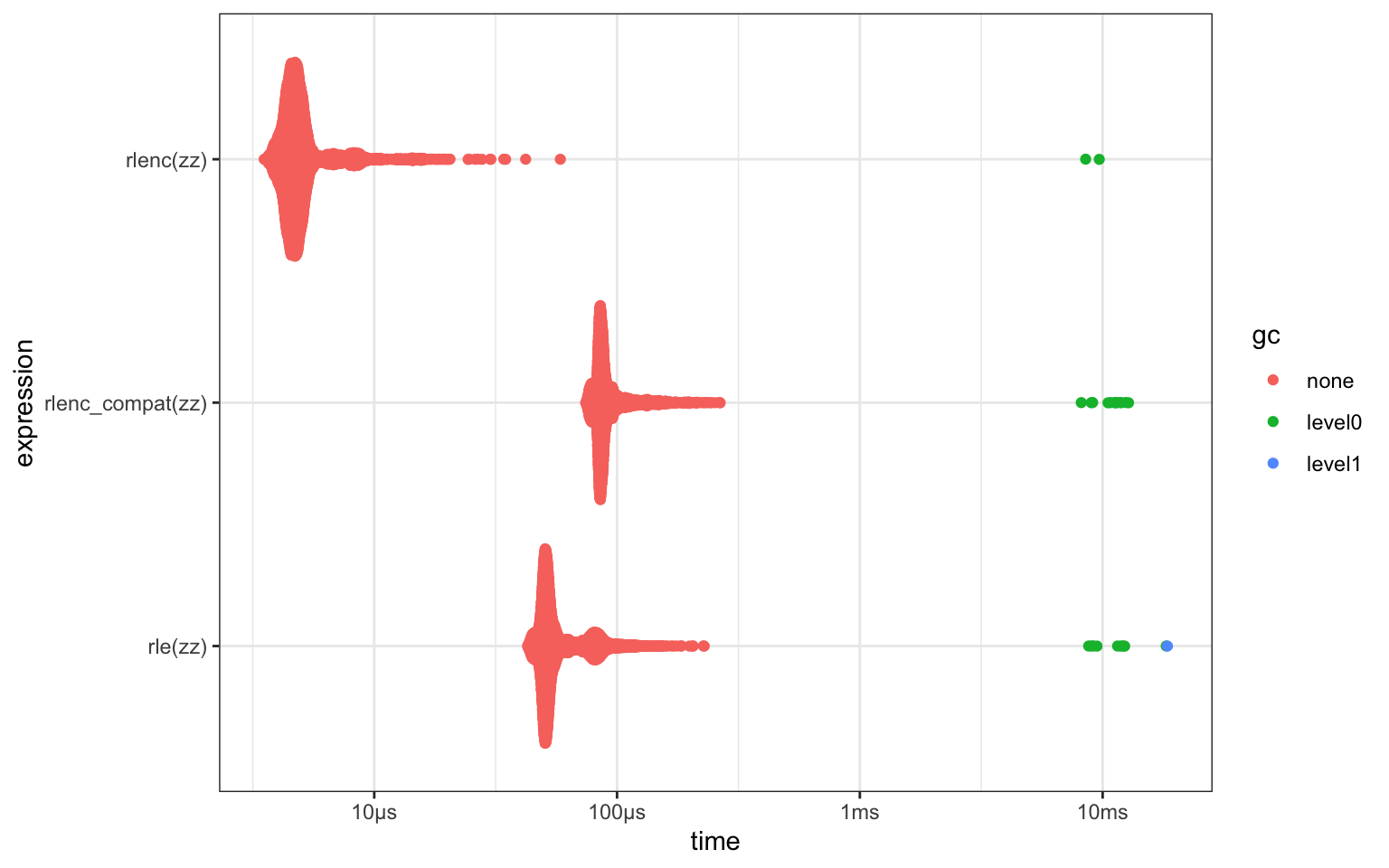# purler`purler` contains tools for run-length encoding vector data.

#### Key features:

• `NA` values are considered identical (unlike `base::rle()`)
• Results returned as a `data.frame` (rather than a list), but still compatible with `base::inverse.rle()`
• Faster! Includes a C implementation for regular atomic vectors, and an R version compatible with every input `base::rle()` accepts.

## What’s in the box

• `rlenc()` is C code for run-length encoding of raw, logical, integer, numeric and character vectors.
• Groups `NA` values into a run (unlike `base::rle()`)
• Returns a data.frame rather than a list
• Returned object is compatible with `base::inverse.rle()`
• Can be 10x faster than `base::rle()`
• `rlenc_compat()`
• A pure R version of `rlenc()` which is compatible with all inputs that `base::rle()` accepts
• `rleid()` returns an integer vector numbering the runs of identical values within a vector of numeric or character data. This is very similar to `data.table::rleid()`, execpt the `data.table()` version is much more configurable and flexible. This version is probably only useful if you wanted to avoid pulling in `data.table` as a dependency.

## Installation

You can install from GitHub with:

``````# install.package('remotes')
remotes::install_github('coolbutuseless/purler')``````

## ToDo

• Long vector support in `rlenc()`

## `rlenc()` - run-length encoding output as a data.frame

``````input <- c(1, 1, 1, 2, 2, 8, 8, 8, 8, 8, NA, NA, NA, NA)

(result <- purler::rlenc(input))``````
``````  lengths values start
1       3      1     1
2       2      2     4
3       5      8     6
4       4     NA    11``````
``inverse.rle(result)``
``   1  1  1  2  2  8  8  8  8  8 NA NA NA NA``

## `rlenc()` benchmark

``````library(tidyr)
library(bench)
library(dplyr)
library(ggplot2)

N <- 1000
M <- 10

zz <- sample(seq_len(M), N, replace = TRUE)

res <- bench::mark(
rle(zz),
rlenc(zz),
rlenc_compat(zz),
check = FALSE
)

plot(res) + theme_bw()``````## Run-length encoding with NAs

In `base::rle()`, runs of NA values are not treated as a group.

All functions in `purler` do treat NAs as identical for the purpose of creating groups

``````input <- c(1, 1, 2, NA, NA, NA, NA, 4, 4, 4)

base::rle(input)``````
``````Run Length Encoding
lengths: int [1:7] 2 1 1 1 1 1 3
values : num [1:7] 1 2 NA NA NA NA 4``````
``purler::rlenc_compat(input)``
``````  lengths values start
1       2      1     1
2       1      2     3
3       4     NA     4
4       3      4     8``````
``purler::rlenc(input)``
``````  lengths values start
1       2      1     1
2       1      2     3
3       4     NA     4
4       3      4     8``````
``purler::rlenc_id(input)``
``  1 1 2 3 3 3 3 4 4 4``

## Run-length encoded group ids

`rlenc_id()` numbers the runs of identical values in a numeric or character vector.

For a more complete approach to this problem, see `data.table::rleid()`

``````input <- c(11, 11, 12, 12, 12, NA, NA, NA, NA)

rlenc_id(input)``````
`` 1 1 2 2 2 3 3 3 3``